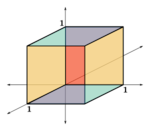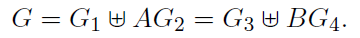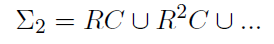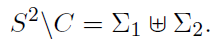# Choose the Axiom

by Carl Pierer

***M.C. Escher: Three Spheres II.

*

If we take an infinite collection of sets, all of which contain at least one element, is there a way to choose exactly one element from each of them? This seems to be just obviously true. After all, there is something in every set, so we can just take any of those things. Certainly, it holds for finite collection of sets. Even if this number is very large, we could just go to each set and pick an element. However, if the collection becomes infinite, this becomes more worrisome. We would need a principled way of choosing one element of each set. Consider, for instance, all non-empty subsets of the natural numbers (the natural numbers being those we use to count: 1, 2, 3, …). By the nature of the natural numbers, such a set will always have a least element. Hence, we could simply pick the least element of each set in our collection. Compare this with an infinite collection of sets of pairs of gloves. As a pair of gloves always consists of a right and a left glove, we can always pick the left glove, say. But this is not so straightforward with other sets. For example, considering all non-empty subsets of the real numbers (containing the natural numbers, but also ¼, e, √π,…) it is far from obvious how we could ensure that we can pick precisely one element from each of those sets. Contrast this with an infinite collection of sets of peas. You know that each set will contain at least 1 pea, but which one are you going to pick if there are more than 1?

The axiom of choice, then, claims that this is always possible. It is, what some may call, somewhat theological, as it asserts the existence of something (a choice function) – even though we might have no idea at all how to construct it, as for instance in the real number example. Yet, it just seems very obvious that this principle should hold. After all, there is something in every set to choose from. The axiom is probably one of the most contentious of the standard axioms of set-theory[i]. On the one hand, it is very powerful and many important proofs in mathematics – explicitly or implicitly – use it. On the other, it leads to some highly counter-intuitive consequences.

It will be seen that thinking about the axiom of choice leads to profound philosophical questions about the nature of mathematical truth and, incidentally, the role of choice. The present essay will attempt to explain one of the most famous and beautiful consequences of the axiom of choice – the Banach-Tarski paradox. In a second essay, the consequences of rejecting the axiom, as well as the philosophical implications of the problematic status of the axiom, will be examined.

*

The axiom of choice, although intuitively sensible, proves to have some rather perplexing consequences. One of the nicest examples is known as the Banach-Tarski Paradox[ii]. The paradox is simple to state: there exists a partitioning of the punctured unit ball into 8 sets and 8 rotation matrices such that: the disjoint union of 4 elements of the partition combined with 4 rotations is equal to the unit ball. The same goes for the remaining elements. In less mathematical terminology: it is possible to break up a ball with its centre missing into 8 pieces in such a way that we can take 4 of those pieces, rotate them around, and get back the original ball. We can do the same thing with the remaining 4 pieces and get another ball of exactly the same size. Thus, by breaking the ball up and rotating it around, we've gotten back twice the original ball! (It was later shown that actually 5 pieces are sufficient)

To prove the Banach-Tarski paradox, we need to show three things:

1. Take all possible rotations in ℝ3 (i.e. “the space we live in”). Then there exists a subgroup (a selection with a special structure) G, such that we can partition G into 4 pieces (break it up such that each element of G is in exactly one of the four pieces): G1, G2, G3, G4. Now, there exist rotations A and B, such that we get back G by combining everything in G1 with everything in G2 rotated by A (that is: G=G1AG2) and similarly for G3 and G4. (This is already somewhat paradoxical, but still digestible)
2. We can remove a countable[iii] subset of points on any sphere and ‘cover up' the resulting ‘holes' by rotating the sphere around.
3. [Hausdorff Paradox] The unit sphere S2 with a countable set of points removed can be broken up into 4 pieces (partitioned), which can then be rotated so that we get back twice the original sphere.

We begin with (1): For illustrative purposes, consider a cube in ℝ3. The set of all possible rotations of the cube define a mathematical group (denoted by SO(3)), that is, they satisfy the following:1. Closed under composition: two rotations applied one after the other give another rotation.
2. There is an identity: not rotating at all, or rotating by 360º around a well-chosen axis, gives back the original orientation of the cube.
3. Every element A has an inverse A-1: Rotating by an angle of φ around a given axis, then rotating around the same axis by –φ gives back the identity.
4. Rotations are associative: A(BC) = (AB)C. Applying first rotation C and then rotation AB is the same as applying rotation BC first and then rotation A.

Now, to show (1), we need to find two rotations A, B, such that their order is infinite. This means, no matter how many times we apply the rotation, we will never get back identity. This can easily be done, but is a bit difficult to visualise in three-dimensions, so let us consider rotations in 2 dimensions. Take a unit square centred at the origin. Now, rotate this square by an irrational degree, let's say: √2/π (in radians). If we rotate it again, the total rotation is: √2/π+√2/π = 2√2/π. More generally, applying the rotation n-times, we have a rotation by n√2/π. The important point to notice here is that for the square to return to its original position, we would need at some point to hit a rotation by 2πk, where k is some natural number. This is because rotating by an integer multiple of is the same as not rotating at all. Hence, for our ‘irrational' rotation to have finite order (i.e. give us back the original orientation of the square), we'd need at some point do the same as not rotate it at all. But this is clearly impossible[iv].

From this, we see that there exists a rotation A in 2-dimensions of infinite order. For brevities sake, let us accept that in 3-dimension there exists another such rotation B, such that no combination of A,A-1,B,B-1 (where neither of the inverses are directly next to each other) gives the identity. Now, let G be the subgroup of SO(3) generated by these four rotations. That is, G is all the possible rotations you can get by applying the four rotations in any order you want.

We call any such string of rotations a `word'. Since each of these rotations has infinite order, the group G will have infinite order, i.e. there are infinitely many such `words'. However, we can definitely give a procedure that will not miss any word (just like counting the natural numbers will never miss any natural number). First, start with the words beginning with A-1. The first few elements in this list will look like this:

1. A-1, A-1A-1, A-1A-1A-1,…
2. A-1B, A-1BA-1, …
3. A-1B-1, A-1B-1A-1,…

Now, the intriguing thing to notice about this list is: it contains almost all of G! To see this, abstract away the leading A-1, then all the words on the list either still begin with an A-1, or they begin with B or B-1. Note, that none of them can begin with A. Hence, this list, with the leading A-1 abstracted gives us all of G, except for those words starting with A. Finally, we can put all of this together to show (1):

1. There exists a partitioning of G into 4 subsets: G1 the words starting with A, G2 the words starting with A-1, G3 the words starting with B, and finally G4 the words starting with B-1.
2. `Abstracting away the leading A-1' in the above argument is equivalent to pre-multiplying by A, for the two cancel each other out.
3. Therefore, (with an exactly analogous argument for B and B-1), we can conclude:This establishes (1) – note that nowhere in the above argument have we used the axiom of choice. Next, we need to show (2): that we can simply remove a countable subset of the sphere and 'cover up' the `holes' by rotating the sphere.The idea here relies heavily on the fact that a sphere S2 in ℝ3 consist of an uncountable set of points. To begin with, let C be a countable set of points on the sphere, i.e. we `simply' count points on the sphere using natural numbers: 1,2,3,… . Now, take a rotation R in ℝ3, that is RSO(3), such that, when we rotate C around with this rotation, we never cover the same elements of C twice. More mathematically speaking, no two elements of C lie in the same R-orbit. In other words, we want to make sure that none of the countably many points gets sent to any other of the countably many points. As there are uncountably many points available where to send them, this is clearly possible, even (countably) infinitely often. Now, we can define a subset of the sphere as all the points our set C get send to, that is:And we define a different subset as everything else, i.e. Σ1 = S22C). Now, it is evident that the sphere with the countable subset C removed is just the disjoint union of these two:But now we can go backwards, too! Take our sphere with the missing subset C, split it up into Σ12 as described above. Rotate Σ2 by R-1 and put the two pieces together. We have recovered the whole sphere: S2 = Σ1R-1Σ2.

This establishes (2). We still haven't used the axiom of choice, but now we turn to (3). To begin with, observe that any rotation in ℝ3 is given by an axis of rotation (a line around which the object is rotated) together with an angle by which we rotate. Now, reconsider the group G we constructed earlier. G is countably infinite. Each element of G defines an axis of rotation. Any such axis intersects the sphere S2 in exactly two points. Let C denote the set of all such intersections for all elements in G. Then C is countably infinite. Furthermore, if we remove C from the sphere (i.e. we consider S2C), we have removed all the points that are kept fixed under the rotations of G. This entails that if p is a point on this new sphere (pS2C), then if two elements of G (i.e. two rotations) send p to the same point, they must be the same rotation. In other words, G acts freely on S2C.

Take any point p S2C. Then there exists some point qS2, such that G(q)= p. Why? Because S2C is the set of all points that are not kept fixed under the rotations of G. This means that if something is in that set, it has to have been rotated there by some element of G. But this means that if we consider the union of all these points p, we regain the entire subset of the sphere S2C. To do so, we need to employ the axiom of choice: for each point pS2C pick a qS2, such that G(q) = p. The axiom here ensures that this is possible (because we are picking one element from an infinite collection of non-empty sets). Then, clearly: S2C = ⊎q ∈ Q G(q) for some QS2.

Hence, we can break our sphere with the fixed points removed (S2C) up into four distinct pieces:But recall, that G = G1AG2 = G3BG4 . If we thus rotate Θ2 (or Θ4) by A (or B, respectively), we have:Obviously, the same goes for Θ3 and Θ4. But this shows the Hausdorff paradox: We can break up the sphere with a countable set of points C removed into 4 pieces, rotate them and get back twice the original sphere (with C removed).

We are almost done with the proof of the Banach-Tarski Paradox. For: we know that we can break up a sphere with some points removed into 4 pieces and recover two spheres of the same size. We know that we can cover up the points that were removed by splitting the sphere in two rotating around a little bit. If we trace this backwards, it is an immediate consequence that we can double the entire sphere by breaking it into 8 pieces. Start with the whole sphere S2. Remove a countable subset of points C to get S2C (this is possible as we've shown). Then, apply Hausdorff's paradox, to get two spheres with the countable set of points missing (in the process, we've have split the sphere into 4 pieces). For each of the spheres, break them in two again (the same pieces as in the first step), rotate them to cover up the missing points and you get back two entire spheres. Counting up the splits, we see that we need to break the sphere into 8 disjoint pieces. An analogous argument works for a solid ball (with, perhaps, the centre point removed) and thus the Banach-Tarski paradox is established.

*

It was shown that the axiom of choice leads to the Banach-Tarski paradox, a consequence that is highly counterintuitive. Indeed, it runs counter a very basic philosophical conviction: nihil ex nihilo. Yet, we have managed, at least in the abstract realm of geometry, to duplicate a sphere. Does this count as a reductio ad absurdum of the axiom of choice? Or is it one of the consequences, an antinomy we have to accept? The second part of this essay, published next month, will venture into the philosophical implications of the paradox.

***

References:

Herrlich, H. (2006). Axiom of Choice. Berlin: Springer.

Maddy, P. (1988). Believing the Axioms. I. The Journal of Symbolic Logic, 481-511.

Stromberg, K. (1979). The Banach-Tarski Paradox. The American Mathematical Monthly, 151-161.

Terence, T. The Banach-Tarski Paradox. Retrieved (13 March 2016) from UCLA Department of Mathematics: math.ucla.edu/~tao/preprints/Expository/banach-tarski.pdf

[i] Maddy writes: “The axiom of choice has easily the most tortured history of all the set-theoretic axioms (…)” (Maddy, 1988)

[ii] A fully rigorous mathematical presentation lies beyond the scope of this essay. As further reading two articles may be suggested:

Tao, Terence: The Banach-Tarski Paradox. (A mathematically more advanced, concise presentation. The present exposition follows this article closely, trying to make it more accessible.)

Stromberg, Karl: The Banach-Tarski Paradox. (Presupposing much less mathematical knowledge than the previous article, more in-depth)

[iii] The distinction between countable and uncountable infinity is due to Cantor, who provided one of the most beautiful proofs in mathematics to show that the reals ℝ are uncountably infinite, whilst the rationals, integers, and naturals all have the same “size'' of infinity, namely countable. For a neat presentation, see here.

[iv] Suppose, for a proof by contradiction, that this was possible. Then, for some n,k ∈ ℕ, n √2/π = 2πk. From this, we get that: √2/π2 = 2k/n. The left-hand side is irrational, whilst the right-hand side is rational, contradiction.